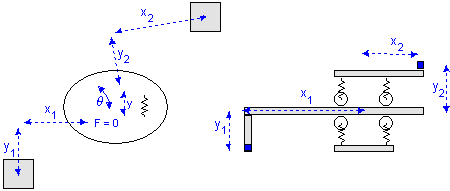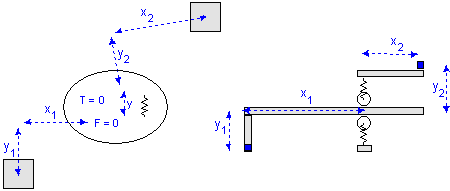﻿ 20-sim webhelp > Library > Iconic Diagrams > Mechanical > Translation > 2DSmallRotation > TwoDLinearSlide

# TwoDLinearSlide

Navigation:  Library > Iconic Diagrams > Mechanical > Translation > 2DSmallRotation >

# TwoDLinearSlide## Library

Iconic Diagrams\Mechanical\Translation\2DSmallAngles

X

Y

XTheta

YTheta

## Use

Domains: Continuous. Size: 2-D. Kind: Iconic Diagrams (2D Planar).

## Description - X

The TwoDLinearSlide-X model represents a flexible connection between two bodies in y- and q-direction. The bodies are located relative to the center of stiffness. The offsets from the bodies to the center of stiffness are indicated by the parameters x1, y1, x2 and y2 as shown in the picture below.

The TwoDLinearSLide-X model is based on the TwoDSpring model by replacing the spring and damper in x-direction by a zero force. Consider a carriage that is clamped with four wheels to a slide. In the x-direction the carriage is free to move, which is equal to a zero force. The limited stiffness of the wheels is represented by springs. If there is a force with equal sign on both wheels, the carriage will slightly move in the y-direction. If there is a force with opposite sign on both wheels, the carriage will only rotate. This can be represented by two independent springs: one in the y-direction and q-direction.Linear slide: x = free, y = stiff, q = stiff.

For a realistic representation of vibration behavior, every spring has a damper in parallel. If you want to turn off the effects of this damper, choose the damping parameters equal to zero. If you want to damp out unwanted vibrations, choose the damping parameters equal to 0.1% to 10% of the spring parameters.

## Interface - X

 Ports Description P Port with three degrees of freedom (x, y, q). Causality fixed force out P Parameters x1 distance in x-direction from center of stiffness to port 1 [m] y1 distance in y-direction from center of stiffness to port 1 [m] x2 distance in x-direction from center of stiffness to port 2 [m] y2 distance in y-direction from center of stiffness to port 2 [m] ky stiffness in y-direction [N/m] kth rotational stiffness [N.m/rad] dy damping in y-direction [N.s/m] dth rotational damping [N.m.s/rad] Initial Values y_initial The initial extension of the spring [m]. theta_initial The initial rotation of the spring [rad].

## Description - Y

The TwoDLinearSlide-Y model is equal to the TwoDLinearSlide-X model except that here the movement is free in the y-direction.

## Interface - Y

 Ports Description P Port with three degrees of freedom (x, y, q). Causality fixed force out P Parameters x1 distance in x-direction from center of stiffness to port 1 [m] y1 distance in y-direction from center of stiffness to port 1 [m] x2 distance in x-direction from center of stiffness to port 2 [m] y2 distance in y-direction from center of stiffness to port 2 [m] kx stiffness in x-direction [N/m] kth rotational stiffness [N.m/rad] dx damping in x-direction [N.s/m] dth rotational damping [N.m.s/rad] Initial Values x_initial The initial extension of the spring [m]. theta_initial The initial rotation of the spring [rad].

## Description - XTheta

The TwoDLinearSlide-XTheta model represents a flexible connection between two bodies in y-direction. The bodies are located relative to the center of stiffness. The offsets from the bodies to the center of stiffness are indicated by the parameters x1, y1, x2 and y2 as shown in the picture below.

The TwoDLinearSLide-X model is based on the TwoDSpring model by replacing the spring and damper in x- and q-direction by a zero force. Consider a carriage that is clamped with two wheels to a slide. In the x-direction the carriage is free to move, which is equal to a zero force. The carriage is also free to rotate, which is equal to a zero torque. The limited stiffness of the wheels is represented by springs. If there is a force on the wheels, the carriage will slightly move in the y-direction.Linear slide: x = free, y = stiff, q = stiff.

For a realistic representation of vibration behavior, every spring has a damper in parallel. If you want to turn off the effects of this damper, choose the damping parameters equal to zero. If you want to damp out unwanted vibrations, choose the damping parameters equal to 0.1% to 10% of the spring parameters.

## Interface - XTheta

 Ports Description P Port with three degrees of freedom (x, y, q). Causality fixed force out P Parameters x1 distance in x-direction from center of stiffness to port 1 [m] y1 distance in y-direction from center of stiffness to port 1 [m] x2 distance in x-direction from center of stiffness to port 2 [m] y2 distance in y-direction from center of stiffness to port 2 [m] ky stiffness in y-direction [N/m] dy damping in y-direction [N.s/m] Initial Values y_initial The initial extension of the spring [m].

## Description - YTheta

The TwoDLinearSlide-YTheta model is equal to the TwoDLinearSlide-XTheta model except that here the movement is free in the y and q-direction.

## Interface - YTheta

 Ports Description P Port with three degrees of freedom (x, y, q). Causality fixed force out P Parameters x1 distance in x-direction from center of stiffness to port 1 [m] y1 distance in y-direction from center of stiffness to port 1 [m] x2 distance in x-direction from center of stiffness to port 2 [m] y2 distance in y-direction from center of stiffness to port 2 [m] kx stiffness in x-direction [N/m] dx damping in x-direction [N.s/m] Initial Values x_initial The initial extension of the spring [m].